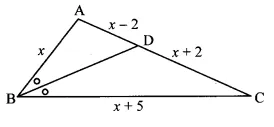# In ∆ABC,seg BD bisects ∠ABC. If AB = x,BC x+ 5, AD = x – 2, DC = x + 2, then find the value of x.

In ∆ABC,seg BD bisects ∠ABC. If AB = x,BC x+ 5, AD = x – 2, DC = x + 2, then find the value of x.verified

x=10